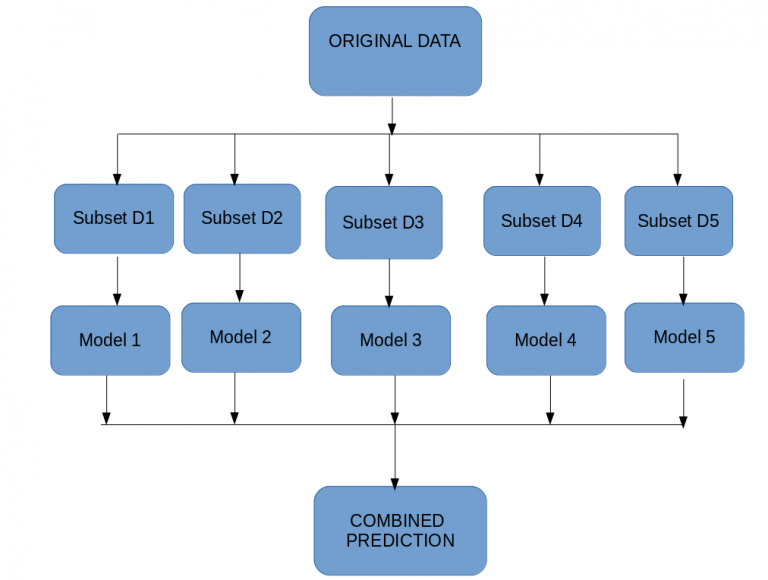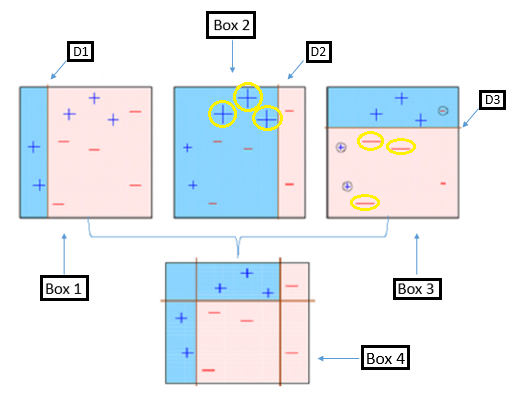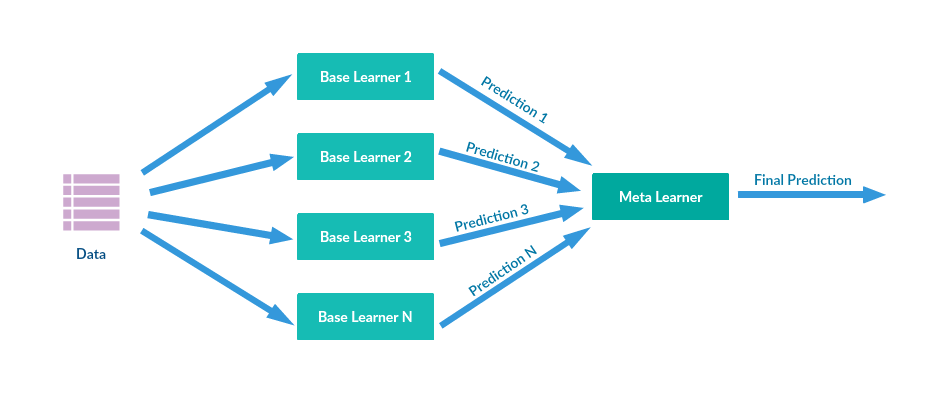×

Search anything:

# Ensemble methods in Machine Learning: Bagging, Boosting and Stacking

#### Machine Learning (ML)In this post, we will be looking at a detailed overview of different Ensemble Methods in Machine Learning. We will see what an ensemble method is, why they are trendy, and what are the different types of ensemble methods and how to implement these methods using scikit-learn and mlxtend in Python.

# What is an Ensemble Method ?

An ensemble method is a Machine Learning technique which utilizes the combined predictions from several machine learning models (also known as base models) into one predictive model to produce more accurate predictions than any single/individual model. By using an ensemble method, we can make better predictions, which in turn improves the performance of our predictive model.

If we observe carefully than ensemble methods are relatable to our real-life scenario. For instance, consider an example of buying a house. If you wish to buy a home, then one possibility could be that you can go to a particular area and directly buy a home from a broker. The second approach which is more realistic could be to go and explore different houses in different areas, get suggestions from our friends and family members, surf over the Internet about reviews of homes and search among all suitable options and than select the best one.

Intuitively, the second approach is much better than the first as we are getting reviews and suggestions from different sources and thus not prone to introducing any biases in our decision making. So, following the second approach, the possibility of purchasing a better house will be much higher. Similarly, ensemble methods combine predictions from learning models in order to produce the most optimal model.

## Types of Ensemble Methods

Now let's look at some of the different Ensemble techniques used in the domain of Machine Learning.

• Bagging
• Boosting
• Stacking

## 1. Bagging

Bagging, also known as Bootstrap Aggregation is an ensemble technique in which the main idea is to combine the results of multiple models (for instance- say decision trees) to get generalized and better predictions. The critical concept in Bagging technique is Bootstrapping, which is a sampling technique(with replacement) in which we create multiple subsets (also known as bags) of observations using the original data. In a nutshell, the approach is:

• Create multiple subsets of original data.
• Train a base model on each subset (each model is independent & runs in parallel to others).
• Final output prediction determined by combining the predictions of each model.Some of the common examples of Bagging models include Bagged Decision Trees, Random Forest, Extra Trees.

These models are very easy to use using Scikit-Learn in Python.

``````from sklearn.ensemble import RandomForestClassifier
from sklearn.metrics import accuracy_score

model = RandomForestClassifier()
model.fit(X_train,Y_train)
pred = model.predict(X_test)

print(accuracy_score(Y_test,pred))
``````

## 2. Boosting

Boosting is a type of ensemble technique which combines a set of weak learners to form a strong learner. As we saw above, Bagging is based on parallel execution of base learners while on the other hand Boosting is a sequential process, wherein each subsequent model attempts to rectify the errors made by the previous model in the sequence which indicates succeeding models are dependent on the previous model.

The terminology 'weak learner' refers to a model which is slightly better than the random guessing model but nowhere close to a good predictive model. In each iteration, a larger weight is assigned to the points which were misclassified in the previous iteration, such that, they are now predicted correctly. The final output in case of classification is computed using weighted majority vote similarly for regression we use the weighted sum.• In Box1, all points are assigned equal weights. A decision stump(parallel to one of the axis) D1 is applied to separate + points from - points.
• In Box2 the points circled in yellow (+) are given higher weights since they were misclassified by D1 and now they correctly classified by D2.
• Similarly in Box3, - points are assigned higher weights since they were misclassified in Box2 by D2.
• Finally, we have Box4 in which all the points are correctly classified. That is why boosting is one of the most powerful ensemble method in Machine Learning.

Some examples of Boosting models include AdaBoost, Stochastic Gradient Boosting, XGBoost etc.

``````from sklearn.ensemble import AdaBoostClassifier
from sklearn.metrics import accuracy_score

model.fit(X_train,Y_train)
pred = model.predict(X_test)

print(accuracy_score(Y_test,pred))
``````

## 3. Stacking

Stacking also referred to Stacked Generalization is an ensemble technique which combines predictions from multiple models to create a new model. The new model is termed as meta-learner. In general, Stacking usually provides a better performance compared to any of the single model. The following figure illustrates the Stacking technique.• We can understand stacking with the help of the following example. Assuming we are splitting our data into three parts- train, validation and test. We are using three different base models SVM, Decision Tree, AdaBoost. We will train each model separately on the training data.
• Now, we will make a meta-learner say Logistic Regression whose inputs will be the predictions by each base model on the validation data so that the meta-learner will be trained on the validation data.
• Now, to finally predict the test data, we will use the meta-learner whose inputs will be the predictions from all the three base models on the test data.

It is straightforward to implement the above example in code. For this, we will require mlxtend along with Scikit-learn.

``````from mlxtend.classifier import StackingClassifier
from sklearn.svm import SVC
from sklearn.tree import DecisionTreeClassifier
from sklearn.linear_model import LogisticRegression
from sklearn.metrics import accuracy_score

model1 = SVC()
model2 = DecisionTreeClassifier()
meta_learner = LogisticRegression()

stack_clf=StackingClassifier(classifiers=[model1,model2,model3], meta_classifier=meta_learner)

stack_clf.fit(X_train,Y_train)
pred = stack_clf.predict(X_test)
print(accuracy_score(Y_test,pred))
``````

## Conclusion

So, in this post, we found what Ensemble Methods are, why they are so powerful, different types of ensemble techniques along with the coding implementation of each. Ensemble techniques are compelling, and in various Machine Learning competitions, you will find that the winning solutions have utilized the ensemble techniques.#### Akshat Maheshwari

Machine Learning Developer, Intern at OpenGenus | Integrated B. Tech and M. Tech student at ABV-Indian Institute of Information Technology and Management (2016 to 2021)

Ensemble methods in Machine Learning: Bagging, Boosting and Stacking